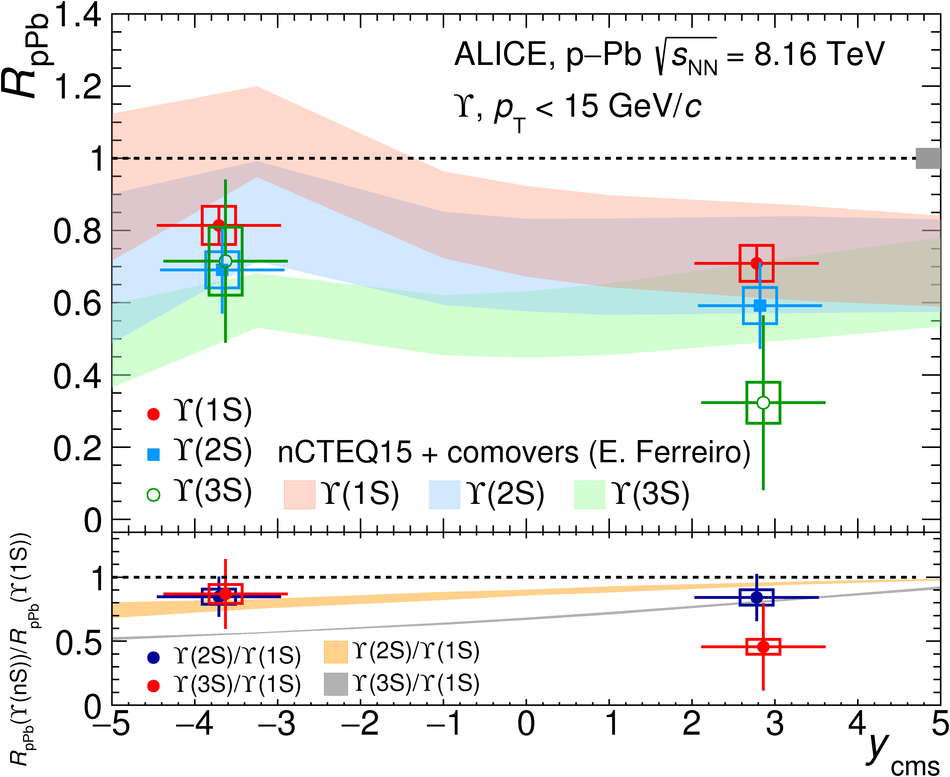# $Υ$ production in p-Pb collisions at $\sqrt{s_{\rm NN}}$ = 8.16 TeV

$\Upsilon$ production in p-Pb interactions is studied at the centre-of-mass energy per nucleon-nucleon collision $\sqrt{s_{\rm{NN}}}$ = 8.16 TeV with the ALICE detector at the CERN LHC. The measurement is performed reconstructing bottomonium resonances via their dimuon decay channel, in the centre-of-mass rapidity intervals $2.03 <~ y_{\rm{cms}} <~ 3.53$ and $-4.46 <~ y_{\rm{cms}} <~ -2.96$, down to zero transverse momentum. In this work, results on the inclusive $\Upsilon(1\rm{S})$ production cross section as a function of rapidity and transverse momentum are presented. The corresponding nuclear modification factor shows a suppression of the $\Upsilon(1\rm{S})$ yields with respect to pp collisions, both at forward and backward rapidity. This suppression is stronger in the low transverse momentum region and shows no significant dependence on the centrality of the interactions. Furthermore, the $\Upsilon(2\rm{S})$ nuclear modification factor is also evaluated, suggesting a suppression similar to that of the $\Upsilon(1\rm{S})$. A first measurement of the $\Upsilon(3\rm{S})$ has also been performed. Finally, results are compared with previous measurements performed by ALICE in p-Pb collisions at $\sqrt{s_{\rm{NN}}}$ = 5.02 TeV and with theoretical calculations.

Figures

## Figure 1

 Invariant mass spectra of unlike-sign dimuons, integrated over \pt, for \Pbp (left panel) and \pPb (right panel) collisions. The shapes of the \upsone, \upstwo and \upsthree resonances are shown (dash-dotted lines), together with the background function (dashed line) and the total fit (solid line).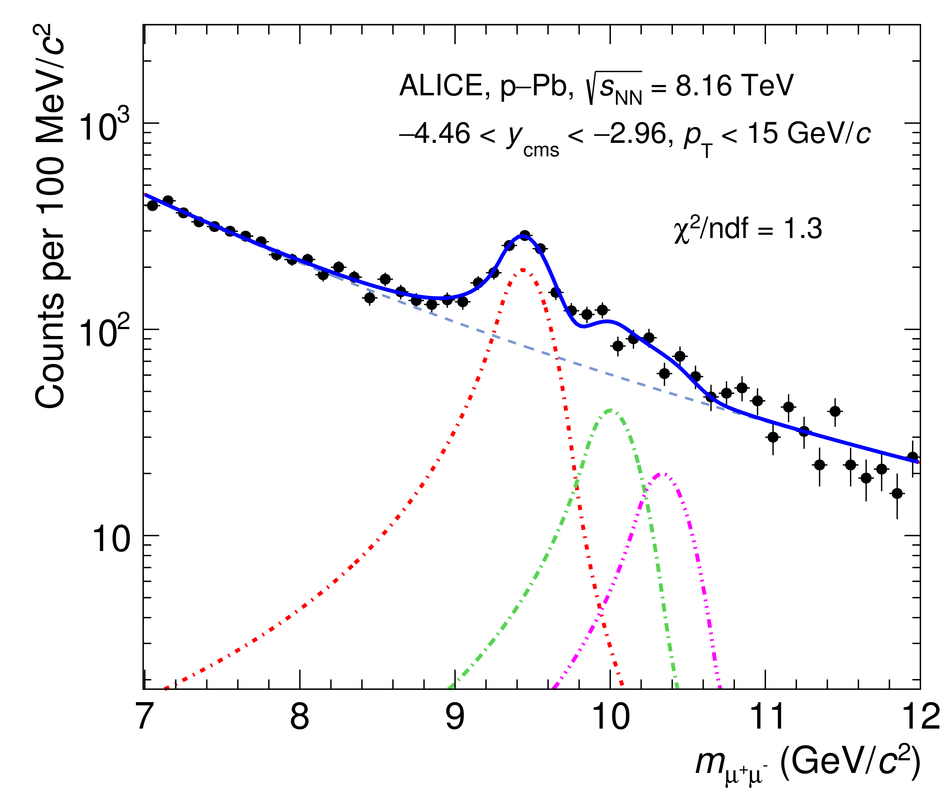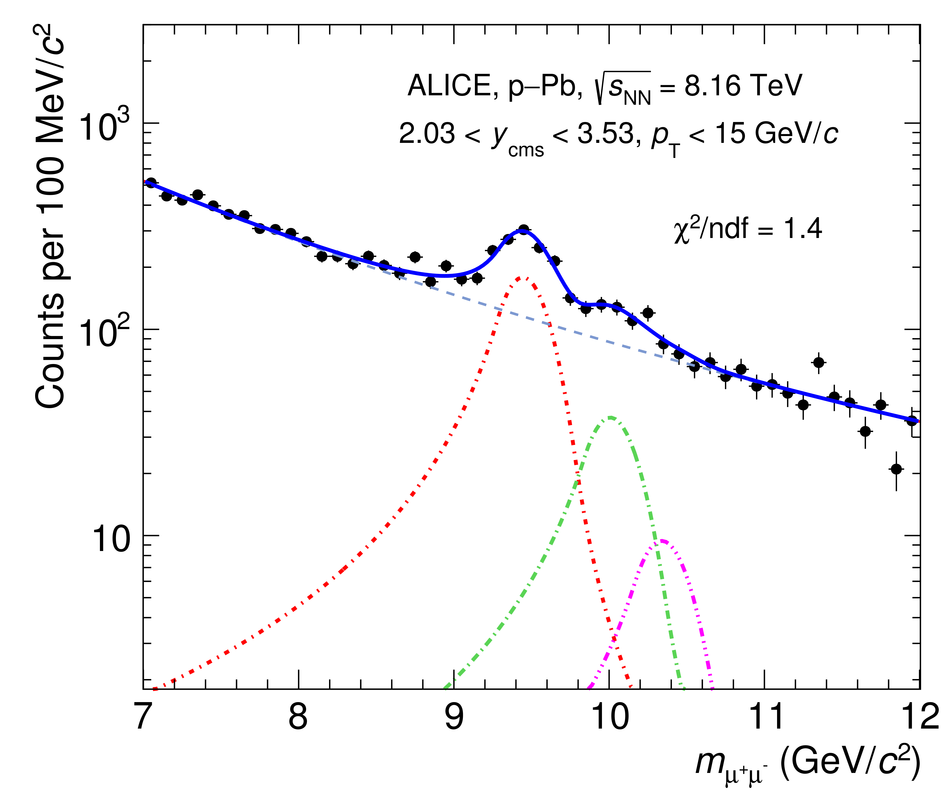## Figure 2

 \upsone, \upstwo and \upsthree differential cross sections as a function of \ycms in \pPb collisions at \sqrts. The corresponding \pp reference cross sections, obtained through the procedure described in Sec.~\ref{sec:analysis} and scaled by $A_{\rm Pb}$, are shown as bands.## Figure 3

 \upsone differential cross section as a function of \pt, at forward (closed symbols) and backward (open symbols) rapidity, at \sqrts. The \pp reference cross section, obtained through the procedure described in Sec.~\ref{sec:analysis} and scaled by $A_{\rm Pb}$, is shown as a band.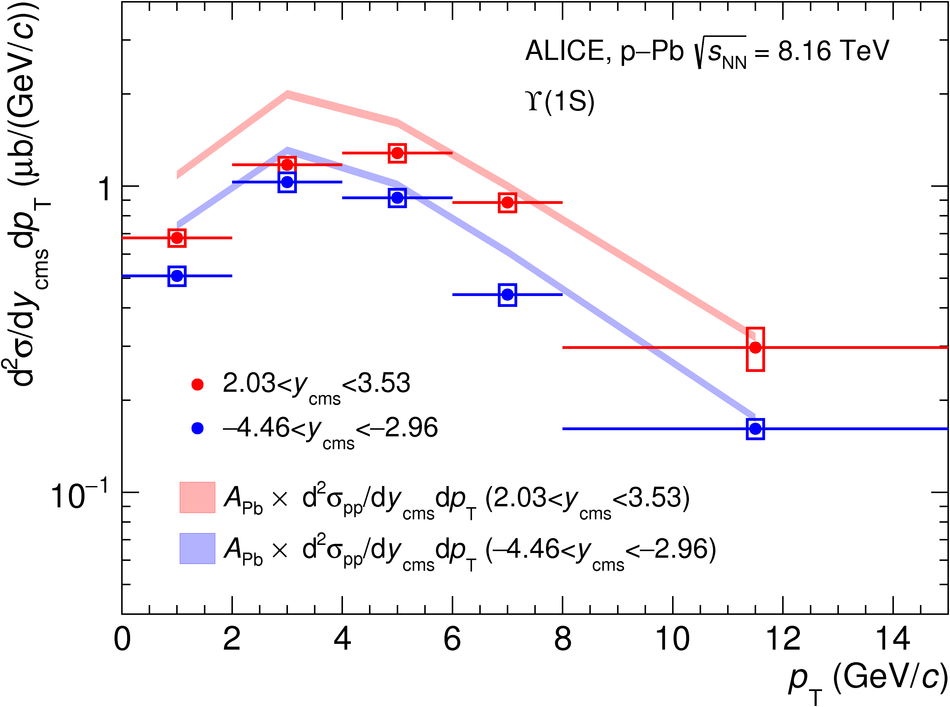## Figure 4

 Ratio of $\Upsilon\rm{(nS)}$ over \upsone yields in \pPb collisions at \sqrts and in \pp collisions at $\sqrt{s}$ = 8 TeV~.## Figure 5

 \upsone \RpPb values at \sqrts compared to those obtained at \sqrtsfive in the same \ycms interval~. All systematic uncertainties are considered as uncorrelated between the results at \sqrts and \sqrtsfive. The \RpPb values at the two energies are slightly displaced horizontally to improve visibility.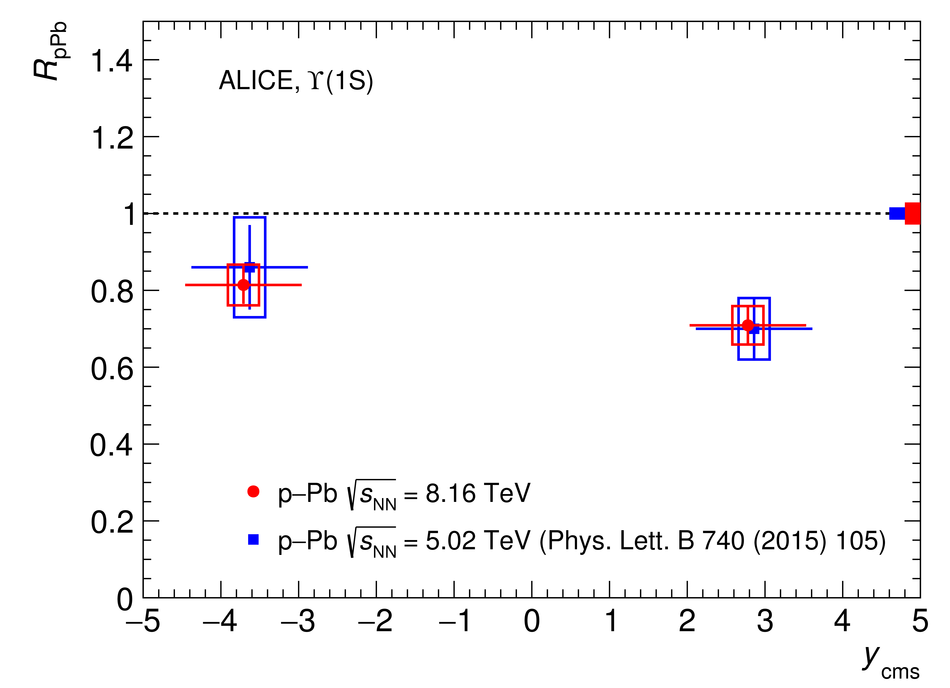## Figure 6

 \upsone \RpPb values at \sqrts compared with the corresponding LHCb results~, as a function of \ycms. The \RpPb values are also compared to model calculations based on several implementations of nuclear shadowing (EPS09 NLO~, EPPS16 and nCTEQ15~) and on parton coherent energy loss predictions, with or without the inclusion of the EPS09 shadowing contribution ~. A theoretical model including a shadowing contribution based on nCTEQ15 nPDFs on top of a suppression induced by comover interactions~ is also shown. For the LHCb results, the vertical error bars represent the quadratic sum of the statistical and systematic uncertainties.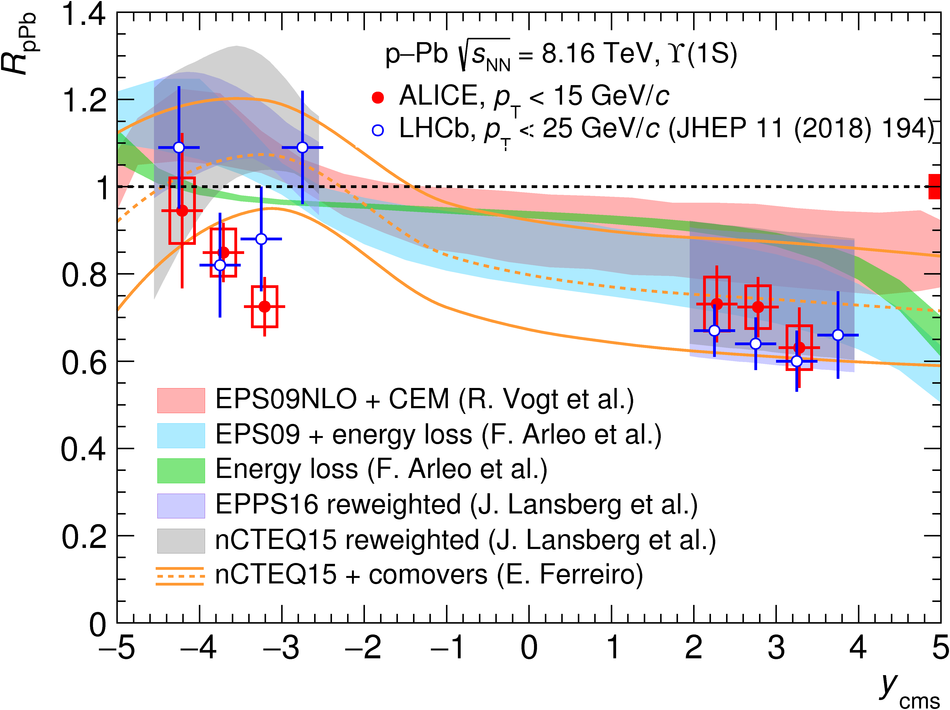## Figure 7

 \upsone \RpPb as a function of \pt for \Pbp (left panel) and \pPb collisions (right panel). The \RpPb values are compared with theoretical calculations based on EPS09 NLO~, nCTEQ15 and EPPS16~ shadowing implementations. Details on the theory uncertainty bands are discussed in the text.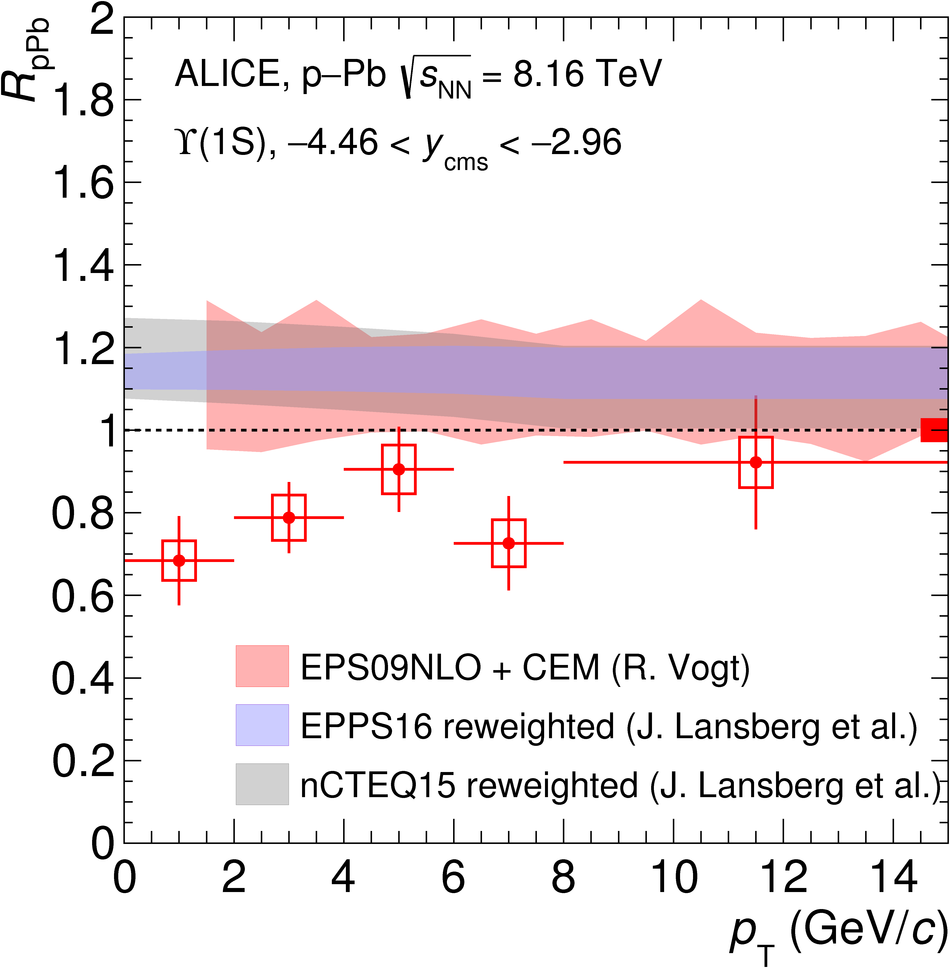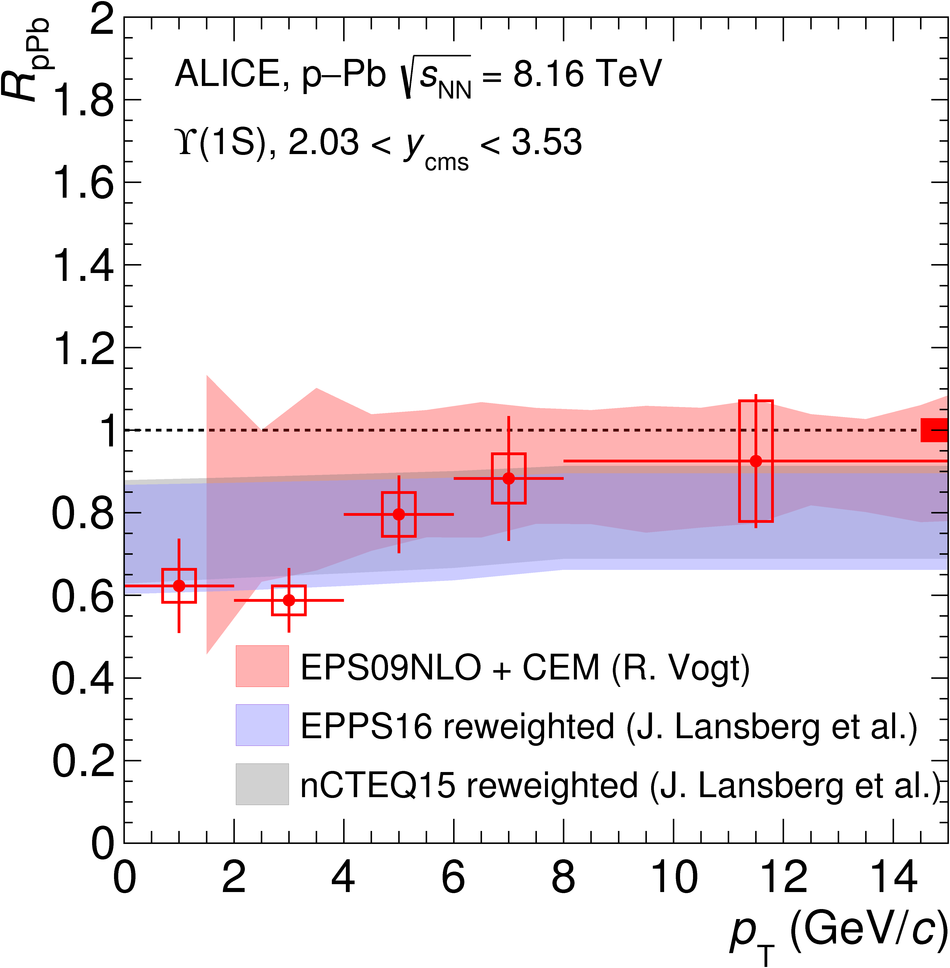## Figure 8

 \upsone \QpPb as a function of \avncoll, for \Pbp (left panel) and \pPb collisions (right panel).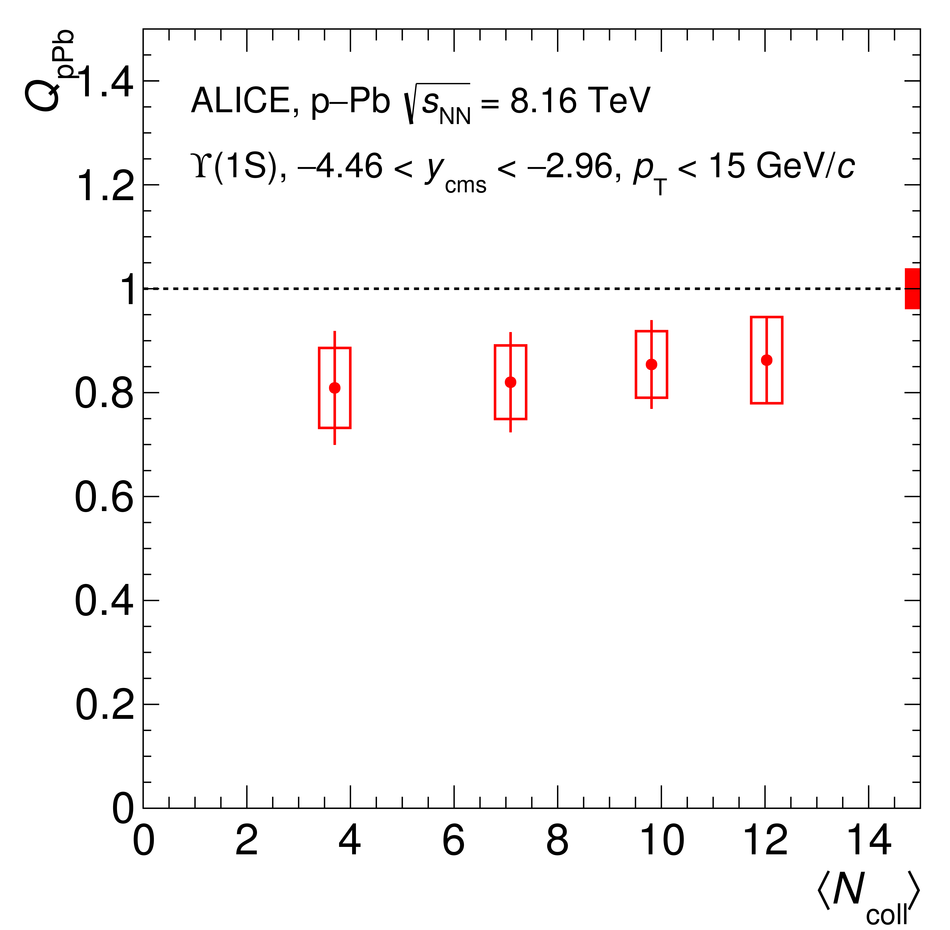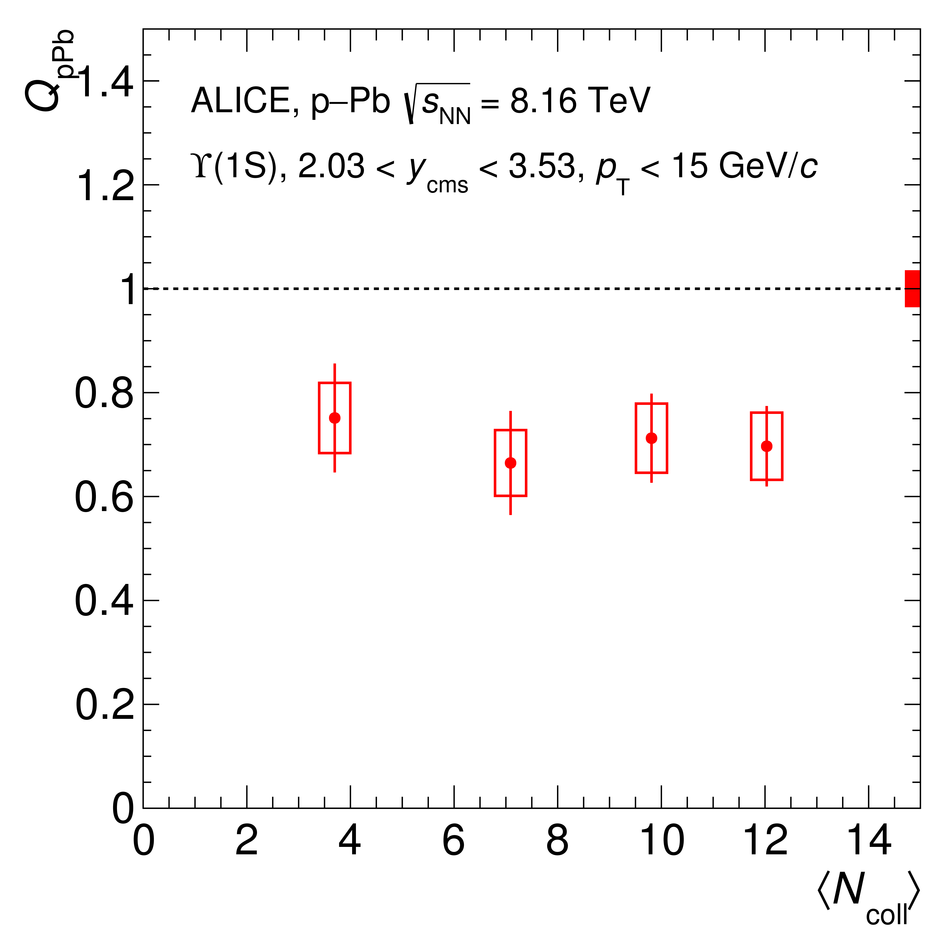## Figure 9

 \upsone, \upstwo and \upsthree \RpPb at \sqrts as a function of \ycms. The \RpPb values of the three resonances are slightly displaced horizontally to improve visibility. Theoretical calculations including nCTEQ15 shadowing contribution and interactions between the \upsi states and comoving particles~ are also shown for all the resonances. The grey box around unity represents the global uncertainty common to the three \upsi states. In the lower panel, the ratio of the \upstwo to \upsone and \upsthree to \upsone \RpPb is shown, together with a calculation based on the aforementioned theory model~.